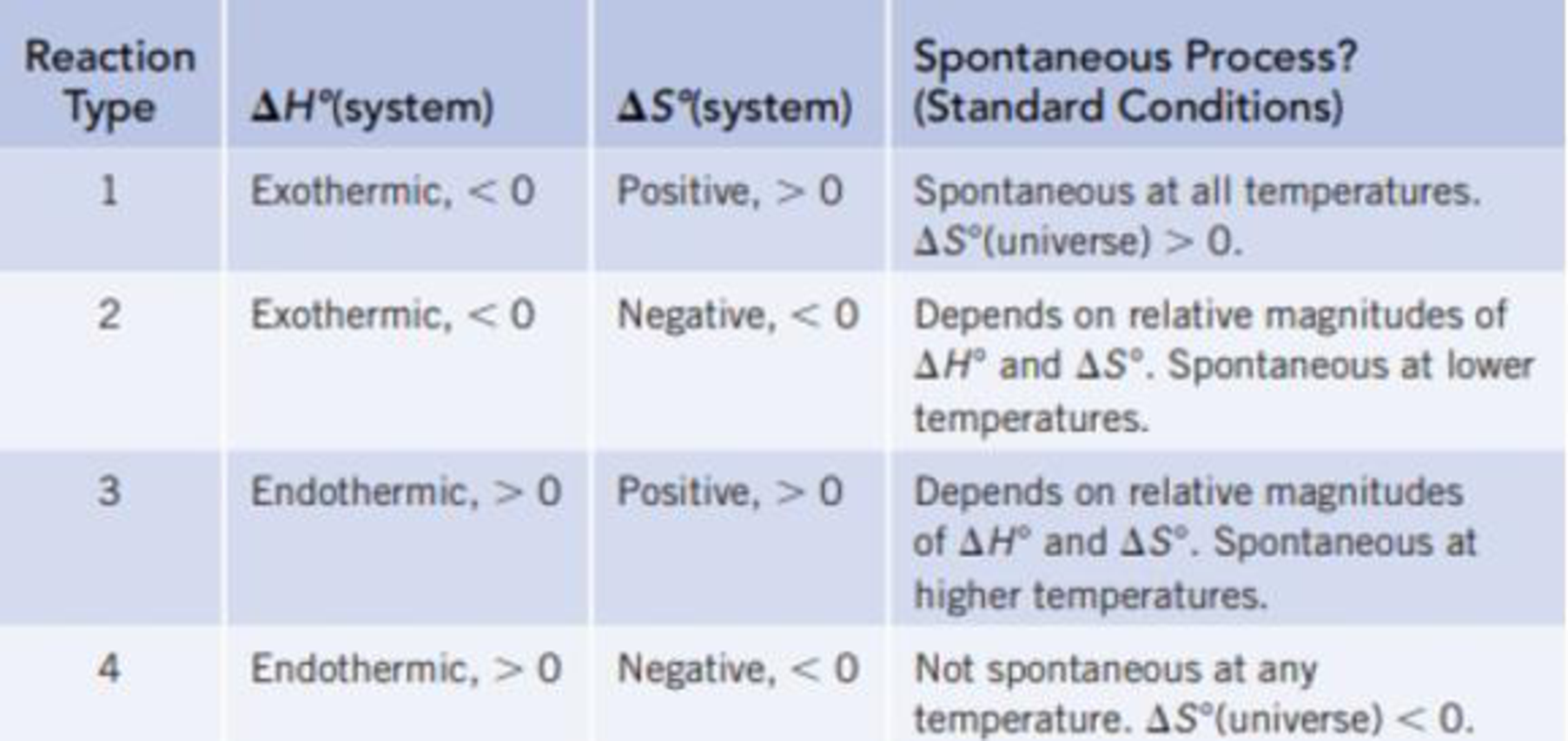# Classify each of the reactions according to one of the four reaction types summarized in Table 18.1. (a) C 6 H 12 O 6 (s) + 6 O 2 (g) → 6 CO 2 (g) + 6 H 2 O( ℓ ) Δ r H ° = −673 kj/mol-rxn Δ r S ° = 60.4 j/K · mol-rxn (b) MgO(s) + C(graphite) → Mg(s) + CO(g) Δ r H ° = 490.7 kJ/mol-rxn Δ r S ° = 197.9 J/K · mol-rxn TABLE 18.1 Predicting Whether a Reaction Will Be Spontaneous Under Standard Conditions### Chemistry & Chemical Reactivity

9th Edition
John C. Kotz + 3 others
Publisher: Cengage Learning
ISBN: 9781133949640

#### Solutions

Chapter
Section### Chemistry & Chemical Reactivity

9th Edition
John C. Kotz + 3 others
Publisher: Cengage Learning
ISBN: 9781133949640
Chapter 18, Problem 14PS
Textbook Problem
172 views

## Classify each of the reactions according to one of the four reaction types summarized in Table 18.1. (a) C6H12O6(s) + 6 O2(g) → 6 CO2(g) + 6 H2O(ℓ) ΔrH° = −673 kj/mol-rxn ΔrS° = 60.4 j/K · mol-rxn (b) MgO(s) + C(graphite) → Mg(s) + CO(g) ΔrH° = 490.7 kJ/mol-rxn ΔrS° = 197.9 J/K · mol-rxn TABLE 18.1 Predicting Whether a Reaction Will Be Spontaneous Under Standard Conditions(a)

Interpretation Introduction

Interpretation:

The reaction C6H12O6(s) + 6O2(g)6CO2(g) + 6H2O(l) should be classified based on reaction types discussed in Table 18.1 on the basis of given ΔS and ΔH values.

Concept introduction:

The universe consists of two parts, systems and surroundings. The entropy change for the universe is the sum of entropy change for the system and for surroundings.

ΔS(universe)= ΔS(system)+ΔS(surroundings)

The ΔS(universe) should be greater than zero for a spontaneous process.

The  ΔS(system) can be calculated by the following expression,

ΔS(system)=ΔrS°=nS°(products)nS°(reactants)

The ΔS(surroundings) can be calculated by the following expression,

ΔS(surroundings)=ΔrHT

Here, ΔrH is the enthalpy change for the reaction.

Thus, a reaction can be categorized as spontaneous or non-spontaneous on the basis of the ΔS and ΔH values.

### Explanation of Solution

The given reaction is,

C6H12O6(s) + 6O2(g)6CO2(g) + 6H2O(l)

The value of ΔrH is 673 kJ/mol- rxn

(b)

Interpretation Introduction

Interpretation:

The reaction MgO(s)+C(graphite)Mg(s)+CO(g) should be classified based on reaction types discussed in Table 18.1 on the basis of given ΔS and ΔH values.

Concept introduction:

The universe consists of two parts, systems and surroundings. The entropy change for the universe is the sum of entropy change for the system and for surroundings.

ΔS(universe)= ΔS(system)+ΔS(surroundings)

The ΔS(universe) should be greater than zero for a spontaneous process.

The  ΔS(system) can be calculated by the following expression,

ΔS(system)=ΔrS°=nS°(products)nS°(reactants)

The ΔS(surroundings) can be calculated by the following expression,

ΔS(surroundings)=ΔrHT

Here, ΔrH is the enthalpy change for the reaction.

Thus, a reaction can be categorized as spontaneous or non-spontaneous on the basis of the ΔS and ΔH values.

### Still sussing out bartleby?

Check out a sample textbook solution.

See a sample solution

#### The Solution to Your Study Problems

Bartleby provides explanations to thousands of textbook problems written by our experts, many with advanced degrees!

Get Started

Find more solutions based on key concepts
T-cells are immune cells that "read" and "remember" chemical messages to identify future invaders. T F

Nutrition: Concepts and Controversies - Standalone book (MindTap Course List)

22-83 Which diseases are associated with amyloid plaques?

Introduction to General, Organic and Biochemistry

What is the function of the central vacuole in plants?

Biology: The Dynamic Science (MindTap Course List)

What causes an aging giant star to produce a planetary nebula?

Horizons: Exploring the Universe (MindTap Course List)

47. Name each alkyne:

Chemistry In Focus

Assign an IUPAC name to each of the following amines.

General, Organic, and Biological Chemistry

Find the equivalent capacitance of a 4.20-F capacitor and an 8.50-F capacitor when they are connected (a) in se...

Physics for Scientists and Engineers, Technology Update (No access codes included)

Anticodons pair with ___ . a. mRNA codons b. DNA codons c. RNA anticodons d. amino acids

Biology: The Unity and Diversity of Life (MindTap Course List)

In what ways did the work of Alfred Thayer Mahan influence the history of the 20th century?

Oceanography: An Invitation To Marine Science, Loose-leaf Versin